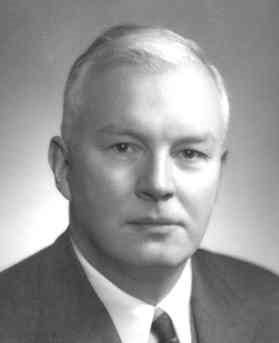# Currying in Calculus, PDEs, Programming, and Category Theory

### Currying is an important concept in both math and functional programming. Let's take a quick look at why this concept is so useful.

· Java Zone · Presentation
Save
7.24K ViewsCurrying is a simple but useful tool in both math and functional programming. It's named after logician Haskell Curry  and has nothing to do with spicy cuisine. If you have a function of two variables, you can think of it as a function of one variable that returns a function of another variable. So, starting with a function f( x, y), we can think of this as a function that takes a number x and returns a function f( x, -) of y. The dash is a placeholder, which can be seen in this recent post.

## Calculus: Fubini's Theorem

If you've taken calculus, then you saw this in the context of Fubini's theorem:To integrate the function of two variables f( x, y), you can temporarily fix y and integrate the remaining function of x. This gives you a number, the value of an integral, for each y, so it's a function of y. You will need to integrate that function, and you have the value of the original function of two variables.

The first time you see this you may think it's a definition, but it's not. You can define the integral on the left directly, and it will equal the result of the two nested integrations on the right or, at least, the two sides will often be equal. The conditions on Fubini's theorem tells you exactly when the two sides are equal.

## PDEs: Evolution Equations

A more sophisticated version of the same trick occurs in partial differential equations. If you have an evolution equation, a PDE for a function on a one-time variable, and several space variables, you can think of it as an ODE via currying. For each time value, t, you get a function of the spatial variables. So, you can think of your solution as a path in a space of functions. The spatial derivatives specify an operator on that space of functions.

I'm glossing over some of the details here because spelling everything out would take a lot of writing and might obscure the big idea. If you'd like the full story, you can check out my graduate advisor's book.

In the Haskell programming language, also named after Haskell Curry, you get currying for free. In fact, there's no other way to express a function of two variables. For example, suppose you want to implement the function f( x, y) = x² + y.

``    Prelude> f x y = x**2 + y``

Then, Haskell thinks of this as a function of one variable (i.e. x) that returns a function of one variable (i.e. f( x, -)), which itself returns a number (i.e. f( x, y)). You can see this by asking the REPL for the type of `f`:

``````    Prelude> :info f
f :: Floating a => a -> a -> a``````

Technically, Haskell, just like lambda calculus, only has functions of one variable. You could create a product datatype consisting of a pair of variables and have your function take that as an argument, but it's still a function on one variable, though that variable is not atomic.

## Category Theory

The way you'd formalize currying in the category theory is to say that the following is a natural isomorphism:For more on what Hom means, check out this post.

## Related Posts

 In concordance with Stigler's law of eponymy, currying was not introduced by Curry but Gottlob Frege. It was then developed by Moses Schönfinkel and developed further by Haskell Curry.

Topics:
functional programming, currying, functions, math, calculus, haskell, java

Published at DZone with permission of John Cook, DZone MVB.

Opinions expressed by DZone contributors are their own.